1597235220

# Momentum ,RMSprop And Adam Optimizer

Optimizer is a technique that we use to minimize the loss or increase the accuracy. We do that by finding the local minima of the cost function.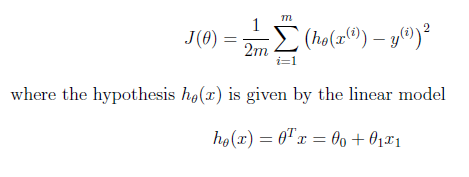Our parameters are updated like this: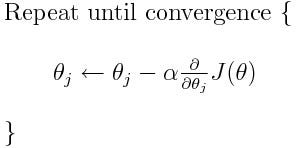When our cost function is convex in nature having only one minima which is its global minima. We can simply use Gradient descent optimization technique and that will converge to global minima after a little tuning in hyper-parameters.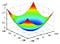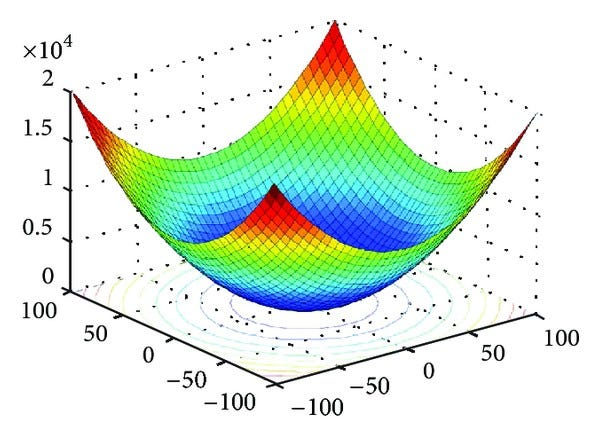But in real world problems the cost function has lots of local minima. And the Gradient Descent technique fails here and we can end up in local minima instead of global minima.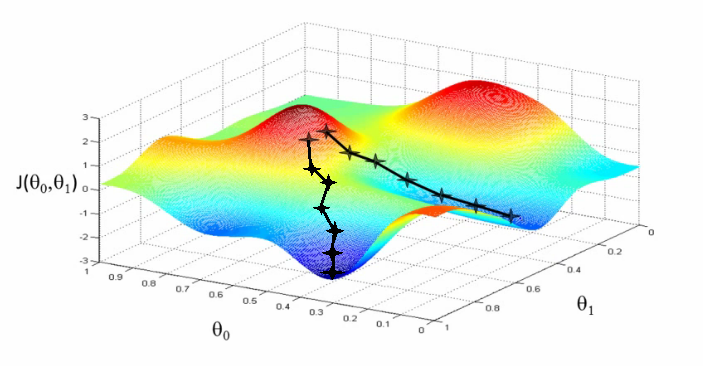So, to save our model from getting stuck in local minima we use an advanced version of Gradient Descent in which we use the momentum.

Imagine a ball, we started from some point and then the ball goes in the direction of downhill or descent. If the ball has the sufficient momentum than the ball will escape from the well or local minima in our cost function graph.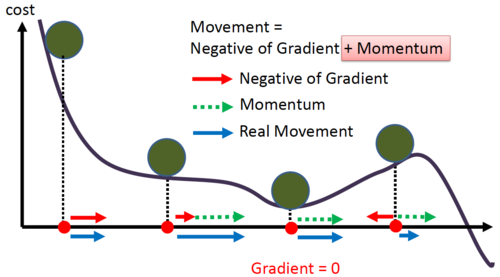Gradient Descent with Momentum considers the past gradients to smooth out the update. It computes an exponentially weighted average of your gradients, and then use that gradient to update the weights.

#neural-networks #deep-learning #optimization-algorithms #algorithms

## Buddha Community1599095520

## Complete Guide to Adam Optimization

In the 1940s, mathematical programming was synonymous with optimization. An optimization problem included an objective function that is to be maximized or minimized by choosing input values from an allowed set of values .

Nowadays, optimization is a very familiar term in AI. Specifically, in Deep Learning problems. And one of the most recommended optimization algorithms for Deep Learning problems is Adam.

Disclaimer: basic understanding of neural network optimization. Such as Gradient Descent and Stochastic Gradient Descent is preferred before reading.

### In this post, I will highlight the following points:

1. Definition of Adam Optimization
3. The Adam Algorithm for Stochastic Optimization
4. Visual Comparison Between Adam and Other Optimizers
5. Implementation
7. Conclusion and Further Reading
8. References

## 1. Definition of Adam Optimization

The Adam algorithm was first introduced in the paper Adam: A Method for Stochastic Optimization  by Diederik P. Kingma and Jimmy Ba. Adam is defined as “a method for efficient stochastic optimization that only requires first-order gradients with little memory requirement” . Okay, let’s breakdown this definition into two parts.

First, stochastic optimization is the process of optimizing an objective function in the presence of randomness. To understand this better let’s think of Stochastic Gradient Descent (SGD). SGD is a great optimizer when we have a lot of data and parameters. Because at each step SGD calculates an estimate of the gradient from a random subset of that data (mini-batch). Unlike Gradient Descent which considers the entire dataset at each step.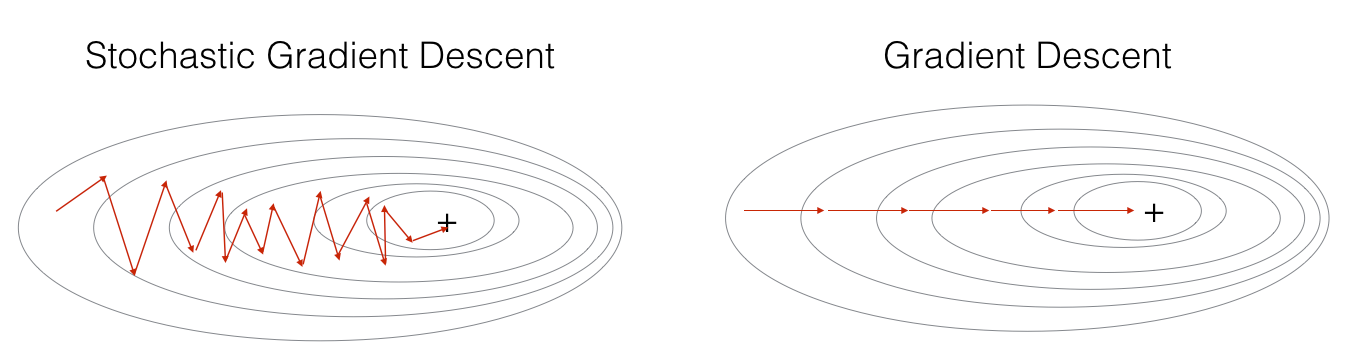#machine-learning #deep-learning #optimization #adam-optimizer #optimization-algorithms1599022440

## Strengths and Weaknesses of Optimization Algorithms Used for ML

Optimization Algorithms for machine learning are often used as a black box. We will study some popular algorithms and try to understand the circumstances under which they perform the best.

### The purpose of this blog is to:

• Understand Gradient descent and its variants, such as _Momentum, Adagrad, RMSProp, NAG, _and Adam;
• Introduce techniques to improve the performance of Gradient descent; and
• Summarize the advantages and disadvantages of various optimization techniques.

Hopefully, by the end of this read, you will find yourself equipped with In with intuitions towards the behavior of different algorithms, and when to use them.1597882980

## Artificial Neural Networks- An intuitive approach Part 5

Contents

2. Autoencoders
3. Dropout
4. Pruning

In this post we will cover a few advanced techniques namely,

A machine learning model can contain millions of parameters or dimensions. Therefore the cost function has to be optimized over millions of dimensions.

The goal is to obtain a global minimum of the function which will give us the best possible values to optimize our evaluation metric with the given parameters.

The odds of obtaining a local minima inmost of the dimensions a high dimensional space are low , we are much more likely to encounter saddle points.

**Saddle Points:**a point at which a function of two variables has partial derivatives equal to zero but at which the function has neither a maximum nor a minimum value.

In mathematics, a saddle point is a point on the surface of the graph of a function where the slopes(derivatives) in orthogonal directions are all zero (a crtitical point), but which is not a local extremum of the function. An example of a saddle point is when there is a critical point with a relative minimum along one axial direction (between peaks) and at a relative maximum along the crossing axis.

Saddle points can drastically slow down optimization process , In the diagrams shown below the stochastic gradient descent converges prematurely to a value which is below optimum. The other points are different optimization techniques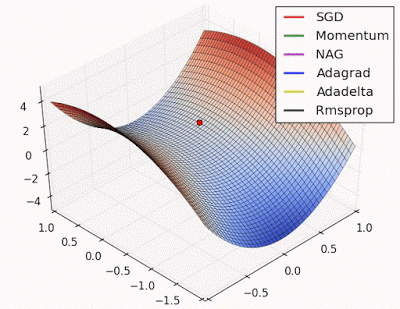In gradient descent we take a step along the gradient in each dimension. In the first animation,using SGD we get stuck in the local minima of one dimension , while we are also at the local maxima of another dimension( Gradient is close to zero)

Because our step size in a given dimension is determined by the gradient value, we’re slowed down in the presence of local optima.

#rmsprop #adam #momentum #autoencoder #dropout1597235220

## Momentum ,RMSprop And Adam Optimizer

Optimizer is a technique that we use to minimize the loss or increase the accuracy. We do that by finding the local minima of the cost function.Our parameters are updated like this:When our cost function is convex in nature having only one minima which is its global minima. We can simply use Gradient descent optimization technique and that will converge to global minima after a little tuning in hyper-parameters.But in real world problems the cost function has lots of local minima. And the Gradient Descent technique fails here and we can end up in local minima instead of global minima.So, to save our model from getting stuck in local minima we use an advanced version of Gradient Descent in which we use the momentum.

Imagine a ball, we started from some point and then the ball goes in the direction of downhill or descent. If the ball has the sufficient momentum than the ball will escape from the well or local minima in our cost function graph.Gradient Descent with Momentum considers the past gradients to smooth out the update. It computes an exponentially weighted average of your gradients, and then use that gradient to update the weights.

#neural-networks #deep-learning #optimization-algorithms #algorithms1624496700

## Optimize Your Algorithms Tail Call Optimization

While writing code and algorithms you should consider tail call optimization (TCO).

### What is tail call optimization?

The tail call optimization is the fact of optimizing the recursive functions in order to avoid building up a tall call stack. You should as well know that some programming languages are doing tail call optimizations.

For example, Python and Java decided to don’t use TCO. While JavaScript allows to use TCO since ES2015-ES6.

Even if you know that your favorite language support natively TCO or not, I would definitely recommend you to assume that your compiler/interpreter will not do the work for you.

### How to do a tail call optimization?

There are two famous methods to do a tail call optimization and avoid tall call stacks.

### 1. Going bottom-up

As you know recursions are building up the call stack so if we avoid such recursions in our algorithms it will will allow us to save on the memory usage. This strategy is called the bottom-up (we start from the beginning, while a recursive algorithm starts from the end after building a stack and works backwards.)

Let’s take an example with the following code (top-down — recursive code):

``````function product1ToN(n) {
return (n > 1) ? (n * product1ToN(n-1)) : 1;
}
``````

As you can see this code has a problem: it builds up a call stack of size O(n), which makes our total memory cost O(n). This code makes us vulnerable to a stack overflow error, where the call stack gets too big and runs out of space.

In order to optimize our example we need to go bottom-down and remove the recursion:

``````function product1ToN(n) {
let result = 1;
for (let num = 1; num <= n; num++) {
result *= num;
}
return result;
}
``````

This time we are not stacking up our calls in the call stack, and we do use a O(1) space complexity(with a O(n) time complexity).

#memoization #programming #algorithms #optimization #optimize